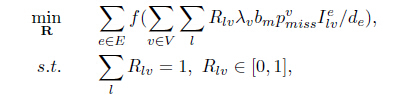# Why is my model wrong?

I try to use the cvx model to solve the problem:and here are my code

cvx_begin
variable R(total_v,2)
for eth=1:total_e
i=1;
for vth=1:total_v
for lth=1:2
u(i)=R(vth,lth)*lamda(vth)bmp_miss(x(vth))I_lve(vth,lth,eth)/de(eth);
i=i+1;
end
end
f(eth)=3
(sum(u))^3;
end
F=sum(f);
minimize(F)
subject to
for i=1:total_v
for j=1:2
R(i,j)>=0&&R(i,j)<=1
end
end
for i=1:total_v
sum(R(i,:))==1
end
cvx_end

The R is variable, and othes have already been setted, and the error message are:
Undefined function or variable ‘op’.

Error in cvx/power>power_p (line 104)
cvx_dcp_error( errs, op );

Error in cvx_binary_op (line 107)
z = p.funcs{vu(1)}( vec(x), vec(y), varargin{:} );

Error in cvx/power (line 31)
z = cvx_binary_op( BP, x, y );

Error in cvx/mpower (line 11)
z = power( x, y, ‘^’ );

Error in test_1 (line 75)
f(eth)=3*(sum(u))^3;
So, what is are the problems in my code?
Thank you.